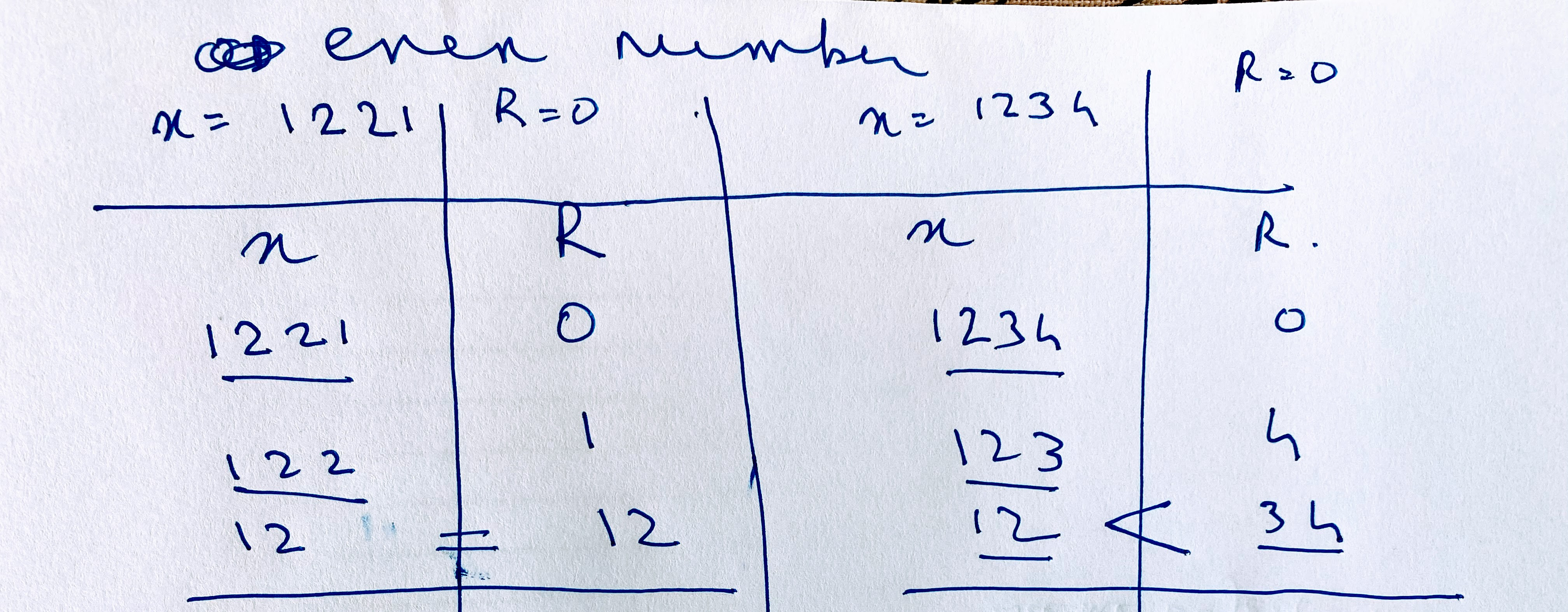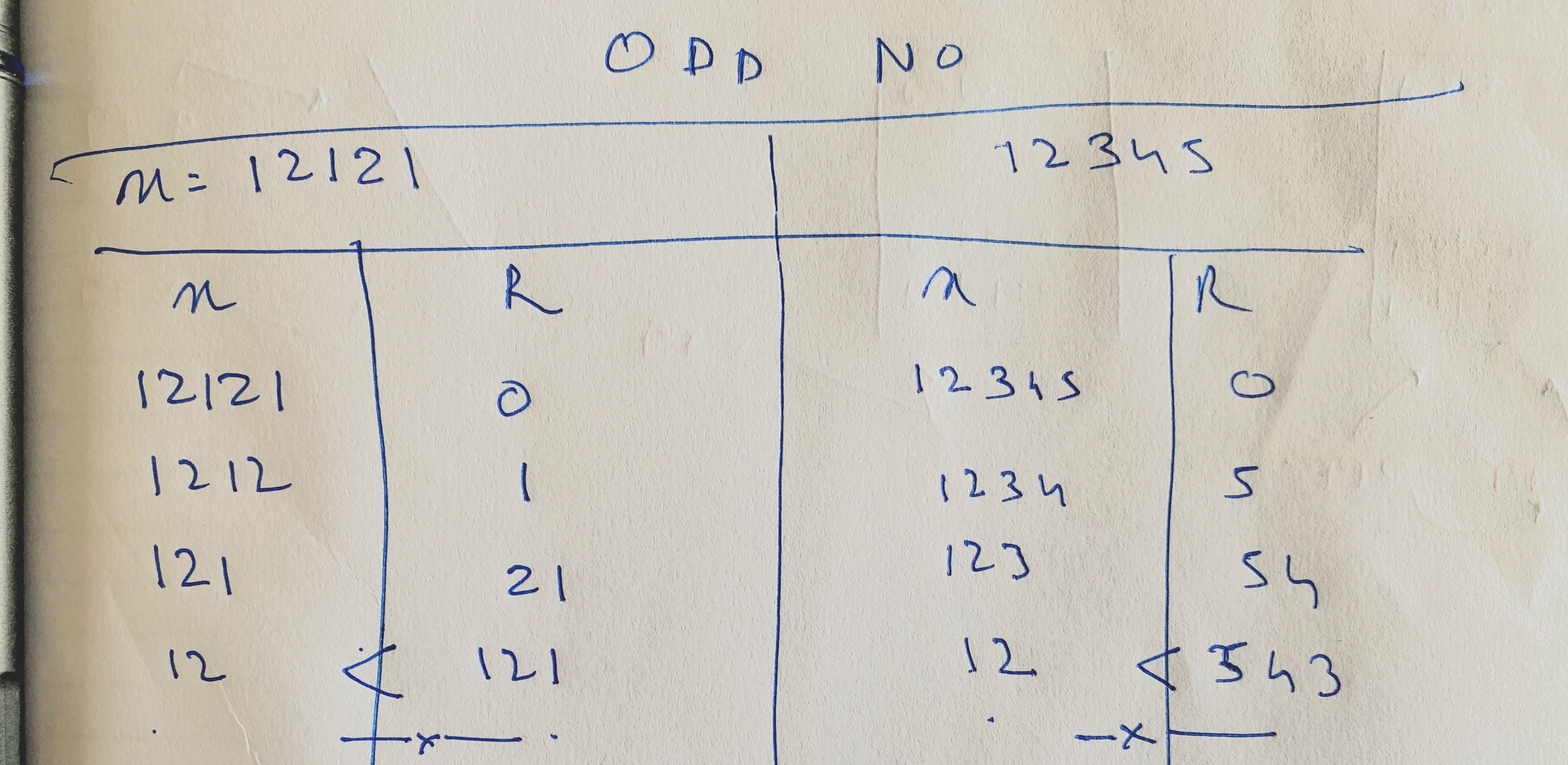# Palindrome

July 20, 2021

In this post, I discuss my experience of solving my very first problem on LeetCode.

## The Problem

To write a program that can check if an integer is a palindrome or not. It was also provided that negative integers and integers ending with one or more zeroes would be deemed to be non-palindromes.

It was suggested that the program be written without converting the integer to a string (though this was not mandatory).

## My Attempt

I solved this problem using a `for-loop`:

``````var isPalindrome = function (x) {
if (x < 0) {
return false;
}
let lastDigit = x % 10;
if (lastDigit == 0) {
if (x == lastDigit) {
return true;
}
return false;
}
let reverse = 0;

for (let i = x; i > 0; i = Math.floor(i / 10)) {
reverse = reverse * 10 + (i % 10);
}
if (reverse == x) {
return true;
}
return false;
};
``````

## Is there a better way to solve this problem?

Although my solution was accepted by LeetCode, I was curious if there was a more elegant solution that took a lesser run-time or required lesser memory to do the same task. So, I looked into some of the solutions posted by others on the discussion section of LeetCode.

Taking a cue from some of the solutions, I figured a better way to write the same program. I realised that the key is to run the loop only till the half-way point.

#### When x is an even number

• First, we start with `reverse = 0` , and `x` is the original value
• At each iteration, we increase `reverse` by the last digit in `x`
• At the same time, we reduce `x` by its last digit, as well
• Thus, at each iteration, `reverse` gains a digit and `x` loses a digit.
• During the course of the loop, there would be three stages, where `x` is a palindrome:
• `x` > `reverse`
• `x` == `reverse`
• `reverse` > `x`
• Now, for non-palindromes, the second step will be skipped. In other words, when both `x` and `reverse` have the same number of digits , they will still be unequal.
• Given this, to determine the mid-point of the integer, we cannot rely on a condition where `x` == `reverse` (because not all `x`s will be palindromes). So we identify the mid-point by seeing when `reverse` becomes either greater than or equal to `x`
• Thus, the loop should run only when `x > reverse`, i.e., it will stop, the moment either `x == reverse` (in case of a palindrome) or `x < reverse`
• Once the loop is terminated, if `reverse` == `x` , then its a palindrome#### When x is an odd number

• Even when `x` is an odd number, the same loop should be used. But note that in odd palindromes, the middle number is not repeated (eg. 12121).
• So, during the iteration of the loop, there will not come a time where the `reverse` and `x` will actually be equal to each other (even when `x` is a palindrome)
• Thus, the point for terminating the loop should be determined by seeing when `x` ceases to be greater than `reverse`. [In the case of odd numbers, this will happen when the `reverse` accumulates more digits than `x`, because there will never be a point when both `reverse` and `x` have the same number of digits]
• After the loop is terminated, to see whether `x` is a palindrome, we will need to ignore the middle number, and then compare the `x` and `reverse`.#### Comparing x with reverse

After the loop is terminated, we should see if `x == reverse || x == Math.Floor(reverse/10`), to see if `x` is in fact a palindrome. [In the case of an odd number, `reverse` will always have an extra digit (i.e., the middle digit). Thus, while comparing the two, we should see if `reverse` without its last digit equals to `x`].

Putting everything together:

``````let reverse = 0;
while (x > reverse) {
reverse = reverse * 10 + (x % 10);
x = Math.floor(x / 10);
}
if (reverse == x || Math.floor(reverse / 10) == x) {
return true;
}
return false;
};
``````